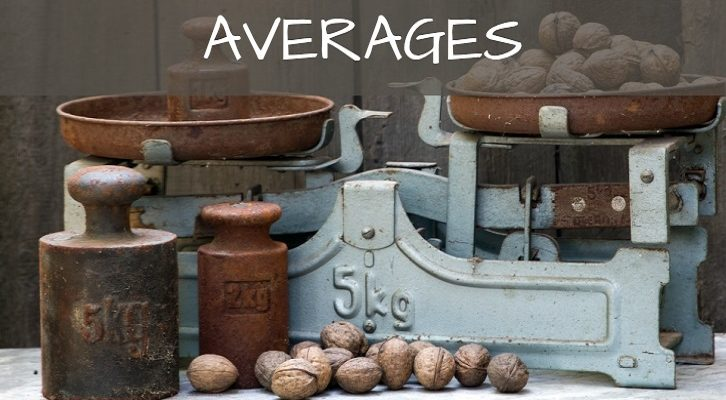# Mean median – Solutions to two more questions

Have given below solutions to the two questions on mean and median.

1. Consider three classes A, B and C with 20, 30 and 50 students respectively. The averages score in maths of students in class B is 16 more than that of those in class C and the average score of those in class A is 2 more than the overall average of scores in A, B and C. What is the difference between average of class C and class A

Let average in class C = x. Average of class B = x +16. Average of class A = y
y = 2 + (20y + 30(x+16) + 50x) /100

(y – 2) * 10 = 2y + 3x + 48 + 5x
10y -20 = 2y + 8x + 48
8y – 8x = 68
y – x = 8.5. This is the difference between average of class A and class C

2. Natural numbers 1 to 25 (both inclusive) are split into 5 groups of 5 numbers each. The medians of these 5 groups are A, B, C, D and E. If the average of these medians is m, what are the smallest and the largest values m can take?

Let us try to construct a scenario for getting the smallest value of the average. We need to have the minimum value for each of the 5 medians.

Now, what is the lowest value the median of any of the 5 groups can take?

The median is the middle term among the 5 terms. So, it cannot be 1 or 2, but it can be 3. If the set were 1, 2, 3, 4, 5, the median would be 3. If we choose a sub-group such as this, the smallest median fro the next group will be 8. The next group could be 6, 7, 8, 9, 10. The idea is to create sub-groups in such a way so that the second group can have a smaller median than 8.

Now, even a sub-group that has the numbers 1, 2, 3, 24, 25 would have the median as 3. So, if we want to keep the medians as small as possible, we should choose sub-groups that have small numbers till the median, and then very large numbers. Because, these large numbers do not affect the median, we will still have small numbers to choose from for the next group. So, choose sub-groups such that the first 3 numbers are small, the final two are as large as possible.

1, 2, 3, 24, 25
4, 5, 6, 22, 23
7, 8, 9, 20, 21
10, 11, 12, 18, 19
13, 14, 15, 16, 17

would be an ideal set of groups. The medians would be 3, 6, 9, 12, 15, and the average of the medians would be 9.

Similar set of groups can be found to find the highest value of the average. The medians would be 23, 20, 17, 14, 11 and the average would be 17.